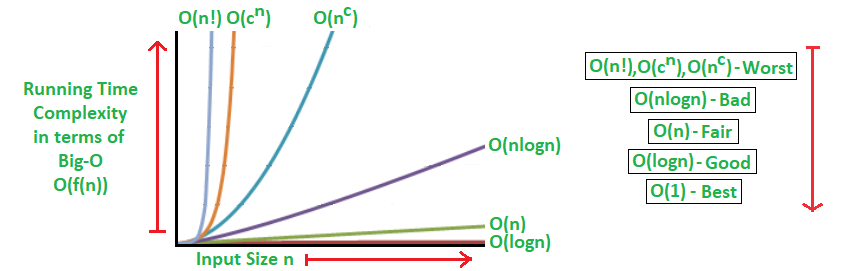# Analysis of Algorithms | Big-O analysis

• Difficulty Level : Medium
• Last Updated : 06 Mar, 2021

In our previous articles on Analysis of Algorithms, we had discussed asymptotic notations, their worst and best case performance etc. in brief. In this article, we discuss the analysis of the algorithm using Big – O asymptotic notation in complete detail.

Big-O Analysis of Algorithms

We can express algorithmic complexity using the big-O notation. For a problem of size N:

• A constant-time function/method is “order 1” : O(1)
• A linear-time function/method is “order N” : O(N)
• A quadratic-time function/method is “order N squared” : O(N 2 )

Definition: Let g and f be functions from the set of natural numbers to itself. The function f is said to be O(g) (read big-oh of g), if there is a constant c > 0 and a natural number n0 such that f (n) ≤ cg(n) for all n >= n0 .

Note: O(g) is a set!

Abuse of notation: f = O(g) does not mean f ∈ O(g).

The Big-O Asymptotic Notation gives us the Upper Bound Idea, mathematically described below:

f(n) = O(g(n)) if there exists a positive integer n0 and a positive constant c, such that f(n)≤c.g(n) ∀ n≥n0

The general step wise procedure for Big-O runtime analysis is as follows:

1. Figure out what the input is and what n represents.
2. Express the maximum number of operations, the algorithm performs in terms of n.
3. Eliminate all excluding the highest order terms.
4. Remove all the constant factors.

Some of the useful properties of Big-O notation analysis are as follow:

▪ Constant Multiplication:
If f(n) = c.g(n), then O(f(n)) = O(g(n)) ; where c is a nonzero constant.
▪ Polynomial Function:
If f(n) = a0 + a1.n + a2.n2 + —- + am.nm, then O(f(n)) = O(nm).
▪ Summation Function:
If f(n) = f1(n) + f2(n) + —- + fm(n) and fi(n)≤fi+1(n) ∀ i=1, 2, —-, m,
then O(f(n)) = O(max(f1(n), f2(n), —-, fm(n))).
▪ Logarithmic Function:
If f(n) = logan and g(n)=logbn, then O(f(n))=O(g(n))
; all log functions grow in the same manner in terms of Big-O.

Basically, this asymptotic notation is used to measure and compare the worst-case scenarios of algorithms theoretically. For any algorithm, the Big-O analysis should be straightforward as long as we correctly identify the operations that are dependent on n, the input size.

Runtime Analysis of Algorithms

In general cases, we mainly used to measure and compare the worst-case theoretical running time complexities of algorithms for the performance analysis.
The fastest possible running time for any algorithm is O(1), commonly referred to as Constant Running Time. In this case, the algorithm always takes the same amount of time to execute, regardless of the input size. This is the ideal runtime for an algorithm, but it’s rarely achievable.
In actual cases, the performance (Runtime) of an algorithm depends on n, that is the size of the input or the number of operations is required for each input item.
The algorithms can be classified as follows from the best-to-worst performance (Running Time Complexity):

▪ A logarithmic algorithm – O(logn)
Runtime grows logarithmically in proportion to n.
▪ A linear algorithm – O(n)
Runtime grows directly in proportion to n.
▪ A superlinear algorithm – O(nlogn)
Runtime grows in proportion to n.
▪ A polynomial algorithm – O(nc
Runtime grows quicker than previous all based on n.
▪ A exponential algorithm – O(cn
Runtime grows even faster than polynomial algorithm based on n.
▪ A factorial algorithm – O(n!)
Runtime grows the fastest and becomes quickly unusable for even
small values of n.

Where, n is the input size and c is a positive constant.Algorithmic Examples of Runtime Analysis
Some of the examples of all those types of algorithms (in worst-case scenarios) are mentioned below:

▪ Logarithmic algorithm – O(logn) – Binary Search.
▪ Linear algorithm – O(n) – Linear Search.
▪ Superlinear algorithm – O(nlogn) – Heap Sort, Merge Sort.
▪ Polynomial algorithm – O(n^c) – Strassen’s Matrix Multiplication, Bubble Sort, Selection Sort, Insertion Sort, Bucket Sort.
▪ Exponential algorithm – O(c^n) – Tower of Hanoi.
▪ Factorial algorithm – O(n!) – Determinant Expansion by Minors, Brute force Search algorithm for Traveling Salesman Problem.

Mathematical Examples of Runtime Analysis
The performances (Runtimes) of different orders of algorithms separate rapidly as n (the input size) gets larger. Let’s consider the mathematical example:

```If n = 10,                  If n=20,
log(10) = 1;                log(20) = 2.996;
10 = 10;                    20 = 20;
10log(10)=10;               20log(20)=59.9;
102=100;                    202=400;
210=1024;                    220=1048576;
10!=3628800;                20!=2.432902e+1818;```

Memory Footprint Analysis of Algorithms

For performance analysis of an algorithm, runtime measurement is not only relevant metric but also we need to consider the memory usage amount of the program. This is referred to as the Memory Footprint of the algorithm, shortly known as Space Complexity.
Here also, we need to measure and compare the worst case theoretical space complexities of algorithms for the performance analysis.
It basically depends on two major aspects described below:

• Firstly, the implementation of the program is responsible for memory usage. For example, we can assume that recursive implementation always reserves more memory than the corresponding iterative implementation of a particular problem.
• And the other one is n, the input size or the amount of storage required for each item. For example, a simple algorithm with a high amount of input size can consume more memory than a complex algorithm with less amount of input size.

Algorithmic Examples of Memory Footprint Analysis: The algorithms with examples are classified from the best-to-worst performance (Space Complexity) based on the worst-case scenarios are mentioned below:

```▪ Ideal algorithm - O(1) - Linear Search, Binary Search,
Bubble Sort, Selection Sort, Insertion Sort, Heap Sort, Shell Sort.
▪ Logarithmic algorithm - O(log n) - Merge Sort.
▪ Linear algorithm - O(n) - Quick Sort.
▪ Sub-linear algorithm - O(n+k) - Radix Sort.```